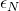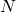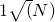Research Interests

UNDER CONSTRUCTIONMean-Field Games (MFGs) is the study of games, whether they are subject to stochastic  dynamics or not, in the limit when the number of agents tends to infinity. MFG formulation of a finite N- player game are often used as approximate solutions. One can often show that when agents use the MFG solution in the finite player game, agents can only improve their value function by an amountwhich tends to zero astends to infinity (usually at the rate of).

Some of my work relates MFGs to algorithmic trading. In electronic trading venues many agents are optimizing against the market. Naturally, their actions affect the market, and, therefore, the action of a single agent affects the rewards/costs of other agents. Hence, trading really is a multi-player game, and we have used MFG techniques to analyze these problems.

Combing agents uncertainty in the underlying model with the optimal actions is another direction that I have been interested in. One line of research looks at developing a general theory of MFGs where agents account for their ambiguity aversion when making optimal decisions.Algorithmic trading generally refers to the automatic trading of assets using a predefined set of rules. These rules can be motivated by financial insights, and/or mathematical and statistical analysis of assets. Price, order-flow, and posted liquidity are often factors in determining how to trade. When decisions are made at ultra-fast time scales, and mostly rely on technological advantages, the strategies are referred to as high-frequency trading strategies.

My research in this arena has focused on the application of stochastic control techniques to pose and solve a variety of algorithmic and high-frequency trading problems.

One example is how to incorporate both limit and market orders into optimal execution problems to reduce the total execution cost. These problems lead to interesting combined optimal stopping and control problems.

Another interesting line of research looks at how to include latent states into the underlying dynamics of the asset prices, while simultaneously trading in an optimal manner.Machine learning (ML) is widely used in a variety of fields where there are rich data sets. Its goal is to allow the data to “speak for itself” using minimal input, or assumptions, from the data scientist. An simple example, stemming from algorithmic trading, is the question of classifying what configurations of the limit order book lead to situations in which the price of asset is more likely to move up versus move down.

My research interest in ML is mostly in the domain of reinforcement learning (RL) as it applies to algorithmic trading. When agents aim to optimize profits, while limiting risks, they are solving a stochastic control problem, since the future dynamics are unknown, and your trading actions affect it an unknown manner. One approach is to assume general models that you then attempt to solve using methods of stochastic analysis and control/stopping. Another, more computational, approach which is somewhat model free is act on the system and see how it reacts, then use the reaction to update what you believe is optimal. This is the essence of RL, and it aims to find optimal actions in a model-free manner.

Being model-free, generally leads to “noisy” optimal actions which don’t have much financial interpretation. My interests lie in tying together stochastic analysis methods with reinforcement learning that lead to results which are financially sound and interpretable.

Content for tab3